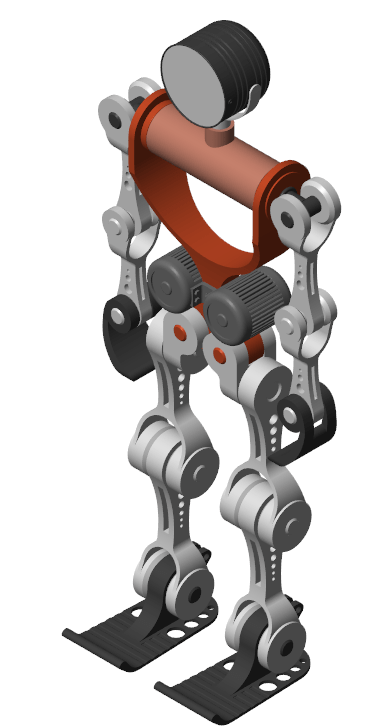# Import a URDF Humanoid Model

You can import a URDF model into the Simscape™ Multibody™ environment. The import process occurs in a single step based on the `smimport` function. The `smimport` function converts the URDF model directly into an equivalent Simscape Multibody model.

### Example Overview

This example shows how to import an Onshape® model of a humanoid robot assembly. The model comprises various parts (”links” in URDF jargon) representing the torso, head, and limbs of the robot. The parts connect through revolute and weld joints (”continuous” and “fixed” respectively).

### Import the Model

To add the URDF file to the search path for the current MATLAB® session, enter:

```openExample("sm/DocImportedURDFModelExample"); addpath(genpath("ImportedURDFSupport")); ```

Import the URDF model:

```smimport("Humanoid.urdf") ```

The function generates a Simscape Multibody model of the humanoid robot. The file extension is required to identify the import file as URDF. Update the imported model (in the Modeling tab, click Update Model.) to open a static visualization in the initial state. The figure shows the results.Build on the model, for example, by adding control systems to actuate the various joints. For a controlled example, at the MATLAB command prompt enter:

```openExample("sm/ImportedURDFExample") ```

Simulate the model to view a simple animation.